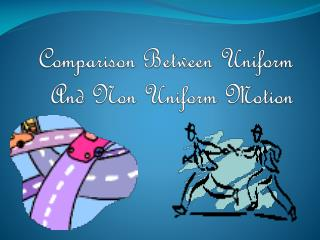# Comparison Between Uniform And Non Uniform Motion - PowerPoint PPT PresentationDownload PresentationComparison Between Uniform And Non Uniform Motion

Comparison Between Uniform And Non Uniform MotionDownload Presentation## Comparison Between Uniform And Non Uniform Motion

- - - - - - - - - - - - - - - - - - - - - - - - - - - E N D - - - - - - - - - - - - - - - - - - - - - - - - - - -
##### Presentation Transcript

1. Comparison Between Uniform And Non Uniform Motion

2. Contents • In this presentation we might see : •  some differences of Uniform and Non Uniform Motion. • Some examples of Uniform and Non Uniform Motion. •  Distance-time graph of Uniform and • Non uniform Motion. •  Velocity-time graph of Uniform and Non Uniform Motion. • Nature of Displacement-time graph of Non uniform Motion.

3. Comparison Between Uniform and Non Uniform Motion :Difference Uniform Motion NonUniform Motion • If an object travels equal distances in equal intervals of time then the object is said to perform uniform motion. • Example1: if a bike covers 10 m in 1st second and another 10 m in 2nd second and continues to travel 10 m /s then the bike is said to travel by uniform speed • If an object travels unequal distances in equal intervals of time then the object is said to be in non uniform motion. • Example1: A car moving in a crowded street.

4. Example2:Uniform Motion- Car A • Example2:Non Uniform Motion- Car B

5. Uniform motion Non Uniform Motion • Car A travels equal distances in equal intervals of time so it is in uniform motion. • Example 3: The movement of the hands of the clock. They move equal distances in equal interval of time. • Car B travels unequal distances in equal intervals of time so it is said to be in non uniform motion. • Example 3: An athlete running in a race.

6. Now we will see examples of Uniform and Non uniform motion with help of pictures

7. Uniform Motion • A bike moving on a straight road with uniform speed. • A ball moving down An inclined plane

8. NonUniform Motion • A car moving with non uniform speed •  A man riding bike on a rough mountain •  

9. Distance time graph for Uniform Motion (Uniform Speed)

10. From the above graph we see that distance-time graph of uniform motion will be looking like a straight line since the objecttravels equal distances in equal intervals of time.

11. Distance-time graph for a Non-Uniform Motion • The following table gives the distance covered by a bus after every 15 minutes. • From the above table we can conclude that the motion is non-uniform i.e., it covers unequal distances in equal intervals of time.

12. Therefore according to the information given in the table , the Distance-Time graph for non uniform motion will be,

13. From the graph in the previous slide we can see that the graph is of oblong shape since it covers unequal distances in equal intervals of time • And from the both distance-time graph of uniform and non uniform motion we conclude that they both differ in shape.

14. Velocity- Time graphs of a car For Uniform And Non Uniform Motion • When an object possesses uniform motion, its velocity-time graph is a straight line parallel to the time axis, as illustrated in the figure below.

15. From the above graph i.e., velocity-time graph, one can calculate the displacement by finding the area of the shaded portion. • displacement in a time t = Area of the rectangle OABC= OA x OC • displacement = velocity x time • Again, in uniform motion, a particle undergoes equal displacement in equal intervals of time

16. Velocity-Time graph of Non Uniform Motion

17. Velocity –Time graph for Non Uniform Motion The velocity-time graph of non uniform motion will be of oblong shape since it has different velocities at different intervals of time .

18. Thus we conclude that velocity time graph of uniform motion and non uniform motion look different from one another as in uniform motion the velocity is the same throughout the time period whereas in v-t graph of non uniform motion velocity and time both increases.

19. Nature of Displacement -time Graph for Non- Uniform Motion • Fig (a) represents the Displacement-Time graph when the speed of a moving object increases and Fig (b) represents the Displacement-Time graph when the speed of a moving object decreases. • From the nature of displacement-time graph we can conclude whether the object is moving with uniform speed or variable speed.

20. The End • Made by • Malay • Nagda